# How to use the Excel TAN function

TAN function is a Mathematical function to calculate the Tangent of the angle of the triangle.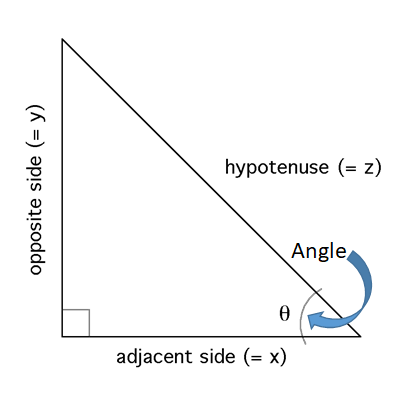Tangent of an angle is the angle made by the adjacent side and opposite to the angle () of the triangle
Tangent(Angle()) = Adjacent side(=x)or base / height(=y)
Syntax:

=TAN (number)

Number in radians or use Excel RADIANS function to convert degrees to radians OR multiply the angle(in degrees) with PI()/180.

Let’s understand this function using it in an example.These are the length of sides of a Triangle having base, height and hypotenuse.

So we need to find the Tangent value of the Angle.
Use the formula:

=TAN(A2/B2)

A2/B2 : Adjacent side of the angle is divided by the opposite side of the angle. This will return a number as an argument to the function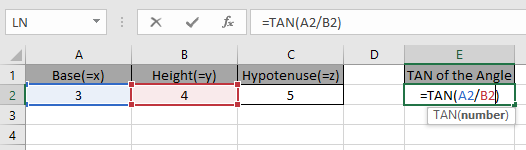Press Enter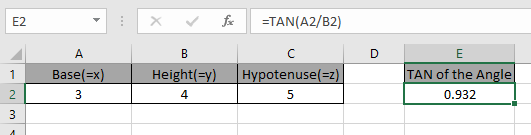As you can see the tangent of the angle of the triangle.

The above method was for having sides of the triangle. If you have an angle in degrees and you need to find the tangent of that angle.

Use the formula:

OR
=TAN(30 * PI()/180)

It will get you the Tan of the 30 degrees angle.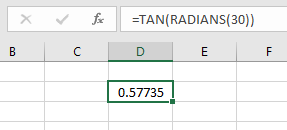Using PI()/180 method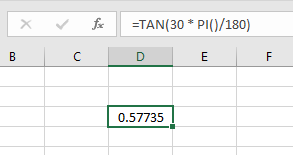As you can see the tangent of the angle using TAN function.

Hope you understood How to use TAN function in Excel. Explore more articles on Mathematical functions like COS and SIN functions here. Please state your query or feedback in the comment box below.

Related Articles:

How to use SIN function in Excel

How to use the Excel COS function

How to use the SINH Function in Excel

How to use the COSH Function in Excel

How to use the TANH Function in Excel

Popular Articles:

How to use the VLOOKUP Function in Excel

How to use the COUNTIF function in Excel 2016

How to Use SUMIF Function in Excel

Terms and Conditions of use

The applications/code on this site are distributed as is and without warranties or liability. In no event shall the owner of the copyrights, or the authors of the applications/code be liable for any loss of profit, any problems or any damage resulting from the use or evaluation of the applications/code.